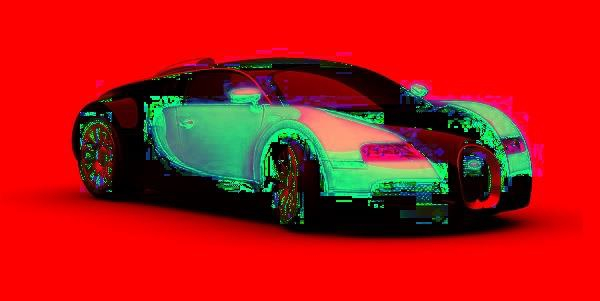# How to convert HSV to colored image using Java OpenCV library?

The cvtColor() method of the Imgproc class changes/converts the color of the image from one to another. This method accepts three parameters −

• src  A Matrix object representing source.

• dst − A Matrix object representing the destination.

• code − An integer value representing the color of the destination image.

To convert an HSV image to RGB you need to pass Imgproc.COLOR_HSV2RGB as the third parameter to this method.

## Example

import org.opencv.core.Core;
import org.opencv.core.Mat;
import org.opencv.imgcodecs.Imgcodecs;
import org.opencv.imgproc.Imgproc;
public class HSV2RGB {
public static void main(String args[]) throws Exception {
//Creating the empty destination matrix
Mat dst = new Mat();
//Converting the image to gray scale
Imgproc.cvtColor(src, dst, Imgproc.COLOR_HSV2RGB);
//Instantiating the Imagecodecs class
Imgcodecs imageCodecs = new Imgcodecs();
//Writing the image
imageCodecs.imwrite("D:\images\hsv2rgb.jpg", dst);
System.out.println("Image Saved");
}
}

## Input## Output